# 3-Way Directional Valve (TL)

3-position directional valve in a thermal liquid network

•Libraries:
Simscape / Fluids / Thermal Liquid / Valves & Orifices / Directional Control Valves

## Description

The 3-Way Directional Valve (TL) block represents a valve with three openings in a thermal liquid network, typically between an actuator, pump, and tank. The valve operation is controlled by a single spool displaced according to the signal at port S. You can set the baseline configuration of your valve by specifying the orifices that are open when the spool moves in the positive direction and negative directions in the Positive spool position open connections and Negative spool position open connections parameters, respectively.

You can set the model for valve opening in the Orifice parameterization parameter as a linear relationship or function of user-provided data, which can be applied to one or all flow paths in the valve.In this configuration, Positive spool position open connections is set to `A-T only`. When the signal at port S moves the spool to a positive position, the path between ports A and T is open to flow. The paths between ports P and A and between ports P and T are closed:In this configuration, Negative spool position open connections is set to `P-A only`. When the signal at port S moves the spool to a negative position, the path between ports P and A is open to flow and the paths between ports T and A and between ports P and T are closed:You can open the path between ports P and T by setting either Positive spool position open connections or Negative spool position open connections to ```P-T only``` or `P-A, A-T, and P-T`.

A flow path can be open in either the positive or negative spool positions, but not both.

### Valve Orifice Parameterizations

The Orifice parameterization sets the method for calculating the valve open area. The calculations are based either on the orifice parameters or tabulated data sets specified in the Model Parameterization tab. The block uses the same data for all flow paths if Area characteristics is set to ```Identical for all flow paths```; otherwise, individual values are applied in the `Different for all flow paths` setting. The orifice parameterizations are:

• `Linear - area vs. spool travel`

The opening area is a linear function of the spool travel distance and the signal received at port S:

`${A}_{orifice}=\frac{\left({A}_{\mathrm{max}}-{A}_{leak}\right)}{\Delta {S}_{\mathrm{max}}}\Delta S+{A}_{leak},$`

where:

• Amax is the Maximum orifice area.

• Aleak is the Leakage area.

• ΔSmax is the Spool travel between closed and open orifice.

• ΔS is the spool travel distance. For flow paths that are open in the positive position:

`$\Delta S={S}_{orifice_\mathrm{max}}-\Delta {S}_{\mathrm{max}}+S,$`

and for flow paths that are open in the negative position:

`$\Delta S={S}_{orifice_\mathrm{max}}+\Delta {S}_{\mathrm{max}}-S,$`

where Sorifice_max is the Spool position at maximum orifice area.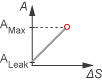When the valve is in a near-open or near-closed position in the linear parameterization, you can maintain numerical robustness in your simulation by adjusting the parameter. If the parameter is nonzero, the block smoothly saturates the opening area between Aleak and Amax. For more information, see Numerical Smoothing.

• `Tabulated data - Area vs. spool travel`

Provide spool travel vectors for your system or for individual flow paths between ports P and A, A and T, and P and T. This data will be used to calculate the relationship between the orifice opening area and spool travel distance. Interpolation is used to determine the opening area between given data points. Aleak and Amax are the first and last parameters of the Opening area vector, respectively.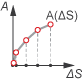• ```Tabulated data - Volumetric flow rate vs. spool travel and pressure drop```

Provide spool travel and pressure drop vectors. The volumetric flow rate is calculated based on the relationship between pressure change and the spool travel distance. Interpolation is used to determine flow rate between given data points. The mass flow rate is the product of the volumetric flow rate and the local density.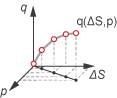When you set Orifice parameterization to ```Tabulated data - Mass flow rate vs. spool travel and pressure drop``` the block calculates the mass flow rate directly from the control member position and the pressure drop across the valve. The relationship between the three variables can be nonlinear and it is given by the tabulated data in the Spool travel vector, ds, Pressure drop vector, dp, and Mass flow rate table, mdot(ds,dp) parameters.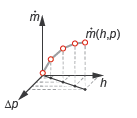### Visualize Orifice Openings

To visualize spool offsets and displacement, right-click the block and select Fluids > Plot Valve Characteristics. The plot shows the orifices selected in the settings. The parameterization selection sets the axes, which are either:

• Orifice area versus spool position

• Volumetric flow rate versus spool position, queried at a specific pressure differential

• Mass flow rate versus spool position for the reference inflow temperature and reference inflow pressure, queried at a specific pressure differential

To update the data after changing the block parameters, click on the figure window.

This image shows an example valve configuration. In the settings:

• Positive spool position open connections is `P-A only`.

• Negative spool position open connections is `A-T only`.

All other spool positions are at the default values.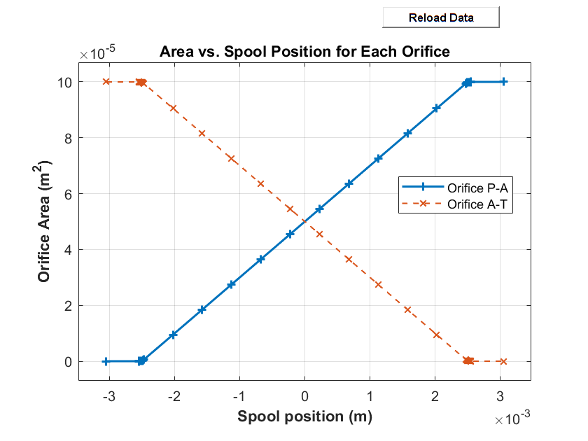### Assumptions

• Fluid inertia is ignored.

• Spool loading due to inertial, spring, and other forces is ignored.

• All valve orifices are assumed to be identical in size unless otherwise specified.

## Ports

### Input

expand all

Displacement of the valve control member, in m. This is equivalent to the spool position.

### Conserving

expand all

Opening through which the flow can enter or exit the valve. This port is typically connected to a fluid supply line.

Opening through which the flow can enter or exit the valve. This port is typically connected to a fluid return line.

Opening through which the flow can enter or exit the valve. This port is typically connected to an actuation line.

## Parameters

expand all

### Model Parameterization

Flow paths open when the spool is in a positive position. This parameter sets the valve configuration and defines the direction of spool movement according to the signal received at port S.

Flow paths open when the spool moves in the negative direction. This parameter sets the valve configuration and defines the direction of spool movement according to the signal received at port S.

Applies uniform or individual flow equations for the valve orifice area. `Identical for all flow paths` uses the same orifice and spool geometries, flow rates, pressure, and area vectors for all valve orifices. When using ```Different for each flow path```, the spool offsets and tabulated data are assigned to the orifices between ports P and A, ports A and T, and ports P and T. In both cases, ports A, P, and T have the same cross-sectional areas, discharge coefficients, and Reynolds numbers. The same Orifice parameterization is also applied to all flow paths.

Method of calculating the valve opening area. In the tabulated data parameterizations, you can provide your own valve area and spool travel data for nonlinear valve opening profiles, or you can provide data in terms of volumetric flow rate, spool travel, and pressure drop over the flow path. The thermal liquid domain allows you to parameterize the orifice using tabulated mass flow rate data.

Maximum distance of spool travel. This value provides an upper limit to calculations so that simulations do not return unphysical values.

#### Dependencies

To enable this parameter, set Area characteristics to ```Identical for all flow paths```.

Largest open area during operation of valve.

#### Dependencies

To enable this parameter, set Area characteristics to ```Identical for all flow paths```.

Vector of control member travel distances for the tabular parameterization of valve area. The vector elements must correspond one-to-one with the elements in the Orifice area vector parameter. The values are listed in ascending order and the first element must be 0. Linear interpolation is employed between table data points.

#### Dependencies

To enable this parameter, set Area characteristics to ```Identical for all flow paths``` and Orifice parameterization to ```Tabulated data - Area vs. spool travel```.

Vector of opening areas for the tabular parameterization of valve opening area. The vector elements must correspond one-to-one with the elements in the Spool travel vector parameter. The elements are listed in ascending order and must be greater than 0.

#### Dependencies

To enable this parameter, set Area characteristics to ```Identical for all flow paths``` and Opening parameterization to ```Tabulated data - Area vs. spool travel```.

Sum of all gaps when the valve is in its fully closed position. Any area smaller than this value is maintained at the specified leakage area. This contributes to numerical stability by maintaining continuity in the flow.

#### Dependencies

To enable this parameter, set Area characteristics to ```Identical for all flow paths``` and Orifice parameterization to ```Linear - area vs. spool travel```.

Continuous smoothing factor that introduces a layer of gradual change based to the flow response when the valve is in near-open and near-closed positions. To increase the stability of your simulation in these regimes, set this parameter to a nonzero value less than one.

Vector of control member travel distances for tabular parametrization of volumetric flow rate. The spool travel vector forms an independent axis with the Pressure drop vector, dp parameter for the 3-D dependent Volumetric flow rate table, q(ds,dp) or Mass flow rate table, mdot(ds,dp) parameters. A positive displacement corresponds to valve opening. The values are listed in ascending order and the first element must be 0. Linear interpolation is employed between table data points.

#### Dependencies

To enable this parameter, set Area characteristics to ```Identical for all flow paths``` and Orifice parameterization to ```Volumetric flow rate vs. spool travel and pressure drop``` or ```Tabulated data - Mass flow rate vs. spool travel and pressure drop```.

Vector of pressure drop values for tabular parametrization of volumetric flow rate. The pressure drop vector forms an independent axis with the Spool travel vector, s parameter for the 3-D dependent Volumetric flow rate table, q(ds,dp) or Mass flow rate table, mdot(ds,dp) parameters. The values are listed in ascending order and must be greater than 0. Linear interpolation is employed between table data points.

#### Dependencies

To enable this parameter, set Area characteristics to ```Identical for all flow paths``` and Orifice parameterization to ```Volumetric flow rate vs. spool travel and pressure drop``` or ```Tabulated data - Mass flow rate vs. spool travel and pressure drop```.

M-by-N matrix of volumetric flow rates based on independent values of pressure drop and spool travel distance. M and N are the sizes of the corresponding vectors:

• M is the number of elements in the parameter.

• N is the number of elements in the Pressure drop vector, dp parameter.

#### Dependencies

To enable this parameter, set Area characteristics to ```Identical for all flow paths``` and Orifice parameterization to ```Tabulated data - Volumetric flow rate vs. spool travel and pressure drop```.

Temperature at which the tabulated pressure-drop data is specified.

#### Dependencies

To enable this parameter, set Orifice parameterization to ```Tabulated data - Mass flow rate vs. spool travel and pressure drop```.

Pressure at which the tabulated pressure-drop data is specified. The block uses this parameter to calculate a third reference parameter, the reference density. The reference that it uses to scale the tabulated pressure drop data for pressures and temperatures deviating from the reference conditions.

#### Dependencies

To enable this parameter, set Orifice parameterization to ```Tabulated data - Mass flow rate vs. spool travel and pressure drop```.

Mass flow rate for M given control member positions and N given pressure drops over the orifice. The control member position represents a spool or other mechanism that controls the opening area.

#### Dependencies

To enable this parameter, set Orifice parameterization to ```Tabulated data - Mass flow rate vs. spool travel and pressure drop```.

Correction factor that accounts for discharge losses in theoretical flows.

Upper Reynolds number limit for laminar flow through the valve.

Select to account for pressure increase when fluid flows from a region of a smaller cross-sectional area to a region of larger cross-sectional area.

Cross-sectional area at the entry and exit ports A, P, and T. These areas are used in the pressure-flow rate equation that determines volumetric flow rate through the valve.

### P-A Orifice

Initial orifice opening distance between ports P and A. The default represents a underlapped system.

• If the magnitude of is less than the value of , the orifice is underlapped, or partially open when the spool position equals 0 m.

• If the magnitude of is greater than the value of , the orifice is overlapped. This means the valve remains closed over a range of spool positions.

• If the magnitude of is equal to the value of , the orifice is zero-lapped. This means the valve is closed when the spool position equals 0 and begins to open as soon as the spool position changes.

Maximum distance of spool travel for the orifice between ports P and A. This value provides an upper limit to calculations so that simulations do not return unphysical values.

#### Dependencies

To enable this parameter, set Area characteristics to ```Different for each flow path```.

Cross-sectional area of the orifice between ports P and A in its fully open position.

#### Dependencies

To enable this parameter, set Area characteristics to ```Different for each flow path``` and Orifice parameterization to ```Linear - area vs. spool travel```.

Vector of control member travel distances for the tabular parameterization of valve area. The vector elements must correspond one-to-one with the elements in the P-A orifice area vector parameter. The values are listed in ascending order and the first element must be 0. Linear interpolation is employed between table data points.

#### Dependencies

To enable this parameter, set Area characteristics to ```Different for each flow path``` and Orifice parameterization to ```Tabulated data - Area vs. spool travel```.

Vector of opening areas for the tabular parameterization of valve opening area. The vector elements must correspond one-to-one with the elements in the P-A orifice spool travel vector parameter. The elements are listed in ascending order and must be greater than 0.

#### Dependencies

To enable this parameter, set Area characteristics to ```Different for each flow path``` and Opening parameterization to ```Tabulated data - Area vs. spool travel```.

Vector of control member travel distances for tabular parametrization of volumetric flow rate. The spool travel vector forms an independent axis with the P-A orifice pressure drop vector, dp parameter for the 3-D dependent P-A orifice volumetric flow rate table, q(s,dp) or Mass flow rate table, mdot(ds,dp) parameters. A positive displacement corresponds to valve opening. The values are listed in ascending order and the first element must be 0. Linear interpolation is employed between table data points.

#### Dependencies

To enable this parameter, set Area characteristics to ```Identical for all flow paths``` and Orifice parameterization to ```Volumetric flow rate vs. spool travel and pressure drop``` or ```Tabulated data - Mass flow rate vs. spool travel and pressure drop```.

Vector of pressure drop values for tabular parametrization of volumetric flow rate. The pressure drop vector forms an independent axis with the P-A orifice spool travel vector, s parameter for the 3-D dependent P-A orifice volumetric flow rate table, q(s,dp) or Mass flow rate table, mdot(ds,dp) parameters. The values are listed in ascending order and must be greater than 0. Linear interpolation is employed between table data points.

#### Dependencies

To enable this parameter, set Area characteristics to ```Identical for all flow paths``` and Orifice parameterization to ```Volumetric flow rate vs. spool travel and pressure drop``` or ```Tabulated data - Mass flow rate vs. spool travel and pressure drop```.

M-by-N matrix of volumetric flow rates based on independent values of pressure drop and spool travel distance. M and N are the sizes of the corresponding vectors:

• M is the number of elements in the P-A orifice pressure drop vector, dp parameter.

• N is the number of elements in the parameter.

#### Dependencies

To enable this parameter, set Area characteristics to ```Different for each flow path``` and Orifice parameterization to ```Tabulated data - Volumetric flow rate vs. spool travel and pressure drop```.

M-by-N matrix of mass flow rates based on independent values of pressure drop and spool travel distance. M and N are the sizes of the corresponding vectors:

• M is the number of elements in the P-A orifice pressure drop vector, dp parameter.

• N is the number of elements in the parameter.

#### Dependencies

To enable this parameter, set Area characteristics to ```Different for each flow path``` and Orifice parameterization to ```Tabulated data - Mass flow rate vs. spool travel and pressure drop```.

### A-T Orifice

Initial orifice opening distance between ports A and T. The default represents a underlapped system.

• If the magnitude of is less than the value of , the orifice is underlapped, or partially open when the spool position equals 0 m.

• If the magnitude of is greater than the value of , the orifice is overlapped. This means the valve remains closed over a range of spool positions.

• If the magnitude of is equal to the value of , the orifice is zero-lapped. This means the valve is closed when the spool position equals 0 and begins to open as soon as the spool position changes.

Maximum distance of spool travel for the orifice between ports A and T. This value provides an upper limit to calculations so that simulations do not return unphysical values.

#### Dependencies

To enable this parameter, set Area characteristics type to ```Different for each flow path```.

Cross-sectional area of the orifice between ports A and T in its fully open position.

#### Dependencies

To enable this parameter, set Area characteristics to ```Different for each flow path``` and Orifice parameterization to ```Linear - area vs. spool travel```.

Vector of control member travel distances for the tabular parameterization of valve area. The vector elements must correspond one-to-one with the elements in the A-T orifice area vector parameter. The values are listed in ascending order and the first element must be 0. Linear interpolation is employed between table data points.

#### Dependencies

To enable this parameter, set Area characteristics to ```Different for each flow path``` and Orifice parameterization to ```Tabulated data - Area vs. spool travel```.

Vector of opening areas for the tabular parameterization of valve opening area. The vector elements must correspond one-to-one with the elements in the A-T orifice spool travel vector parameter. The elements are listed in ascending order and must be greater than 0.

#### Dependencies

To enable this parameter, set Area characteristics to ```Different for each flow path``` and Opening parameterization to ```Tabulated data - Area vs. spool travel```.

Vector of control member travel distances for tabular parametrization of volumetric flow rate. The spool travel vector forms an independent axis with the A-T orifice pressure drop vector, dp parameter for the 3-D dependent A-T orifice volumetric flow rate table, q(ds,dp) or Mass flow rate table, mdot(ds,dp) parameters. A positive displacement corresponds to valve opening. The values are listed in ascending order and the first element must be 0. Linear interpolation is employed between table data points.

#### Dependencies

To enable this parameter, set Area characteristics to ```Identical for all flow paths``` and Orifice parameterization to ```Volumetric flow rate vs. spool travel and pressure drop``` or ```Tabulated data - Mass flow rate vs. spool travel and pressure drop```.

Vector of pressure drop values for tabular parametrization of volumetric flow rate. The pressure drop vector forms an independent axis with the A-T orifice spool travel vector, s parameter for the 3-D dependent A-T orifice volumetric flow rate table, q(ds,dp) or Mass flow rate table, mdot(ds,dp) parameters. The values are listed in ascending order and must be greater than 0. Linear interpolation is employed between table data points.

#### Dependencies

To enable this parameter, set Area characteristics to ```Identical for all flow paths``` and Orifice parameterization to ```Volumetric flow rate vs. spool travel and pressure drop``` or ```Tabulated data - Mass flow rate vs. spool travel and pressure drop```.

M-by-N matrix of volumetric flow rates based on independent values of pressure drop and spool travel distance. M and N are the sizes of the corresponding vectors:

• M is the number of elements in the A-T orifice pressure drop vector, dp parameter.

• N is the number of elements in the parameter.

#### Dependencies

To enable this parameter, set Area characteristics to ```Different for each flow path``` and Orifice parameterization to ```Tabulated data - Volumetric flow rate vs. spool travel and pressure drop```.

M-by-N matrix of mass flow rates based on independent values of pressure drop and spool travel distance. M and N are the sizes of the corresponding vectors:

• M is the number of elements in the A-T orifice pressure drop vector, dp parameter.

• N is the number of elements in the parameter.

#### Dependencies

To enable this parameter, set Area characteristics to ```Different for each flow path``` and Orifice parameterization to ```Tabulated data - Mass flow rate vs. spool travel and pressure drop```.

### P-T Orifice

Initial orifice opening distance between ports P and T. The default represents a underlapped system.

• If the magnitude of is less than the value of , the orifice is underlapped, or partially open when the spool position equals 0 m.

• If the magnitude of is greater than the value of , the orifice is overlapped. This means the valve remains closed over a range of spool positions.

• If the magnitude of is equal to the value of , the orifice is zero-lapped. This means the valve is closed when the spool position equals 0 and begins to open as soon as the spool position changes.

Maximum distance of spool travel for the orifice between ports P and T. This value provides an upper limit to calculations so that simulations do not return unphysical values.

#### Dependencies

To enable this parameter, set Area characteristics type to ```Different for each flow path```.

Cross-sectional area of the orifice between ports P and T in its fully open position.

#### Dependencies

To enable this parameter, set Area characteristics to ```Different for each flow path``` and Orifice parameterization to ```Linear - area vs. spool travel```.

Vector of control member travel distances for the tabular parameterization of valve area. The vector elements must correspond one-to-one with the elements in the P-T orifice area vector parameter. The values are listed in ascending order and the first element must be 0. Linear interpolation is employed between table data points.

#### Dependencies

To enable this parameter, set Area characteristics to ```Different for each flow path``` and Orifice parameterization to ```Tabulated data - Area vs. spool travel```.

Vector of opening areas for the tabular parameterization of valve opening area. The vector elements must correspond one-to-one with the elements in the P-T orifice spool travel vector parameter. The elements are listed in ascending order and must be greater than 0.

#### Dependencies

To enable this parameter, set Area characteristics to ```Different for each flow path``` and Opening parameterization to ```Tabulated data - Area vs. spool travel```.

Vector of control member travel distances for tabular parametrization of volumetric flow rate. The spool travel vector forms an independent axis with the P-T orifice pressure drop vector, dp parameter for the 3-D dependent P-T orifice volumetric flow rate table, q(ds,dp) or Mass flow rate table, mdot(ds,dp) parameters. A positive displacement corresponds to valve opening. The values are listed in ascending order and the first element must be 0. Linear interpolation is employed between table data points.

#### Dependencies

To enable this parameter, set Area characteristics to ```Identical for all flow paths``` and Orifice parameterization to ```Volumetric flow rate vs. spool travel and pressure drop``` or ```Tabulated data - Mass flow rate vs. spool travel and pressure drop```.

Vector of pressure drop values for tabular parametrization of volumetric flow rate. The pressure drop vector forms an independent axis with the P-T orifice spool travel vector, ds parameter for the 3-D dependent P-T orifice volumetric flow rate table, q(ds,dp) or Mass flow rate table, mdot(ds,dp) parameters. The values are listed in ascending order and must be greater than 0. Linear interpolation is employed between table data points.

#### Dependencies

To enable this parameter, set Area characteristics to ```Identical for all flow paths``` and Orifice parameterization to ```Volumetric flow rate vs. spool travel and pressure drop``` or ```Tabulated data - Mass flow rate vs. spool travel and pressure drop```.

M-by-N matrix of volumetric flow rates based on independent values of pressure drop and spool travel distance. M and N are the sizes of the corresponding vectors:

• M is the number of elements in the P-T orifice pressure drop vector, dp parameter.

• N is the number of elements in the parameter.

#### Dependencies

To enable this parameter, set Area characteristics to ```Different for each flow path``` and Orifice parameterization to ```Tabulated data - Volumetric flow rate vs. spool travel and pressure drop```.

M-by-N matrix of mass flow rates based on independent values of pressure drop and spool travel distance. M and N are the sizes of the corresponding vectors:

• M is the number of elements in the P-T orifice pressure drop vector, dp parameter.

• N is the number of elements in the parameter.

#### Dependencies

To enable this parameter, set Area characteristics to ```Different for each flow path``` and Orifice parameterization to ```Tabulated data - Mass flow rate vs. spool travel and pressure drop```.

## Version History

Introduced in R2016a

expand all